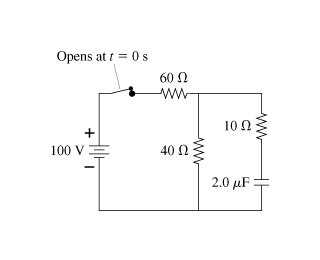# Problem: The switch in the figure has been closed for a very long time.Part A What is the charge on the capacitor?Part B The switch is opened at . t= 0 s At what time has the charge on the capacitor decreased to 29% of its initial value?

###### FREE Expert Solution

Part A

The switch has been closed for long, so the capacitor behaves as an open circuit. No current is flowing from the capacitor.  Equivalent resistance for the series connection:

Req = 60 + 40 = 100 Ω

Current, i = V/R = 100V/100  = 1.0 A

The voltage across the 40Ω - resistor:

98% (113 ratings)###### Problem Details

The switch in the figure has been closed for a very long time.

Part A

What is the charge on the capacitor?

Part B

The switch is opened at . t= 0 s At what time has the charge on the capacitor decreased to 29% of its initial value?Frequently Asked Questions

What scientific concept do you need to know in order to solve this problem?

Our tutors have indicated that to solve this problem you will need to apply the !! Resistor-Capacitor Circuits concept. If you need more !! Resistor-Capacitor Circuits practice, you can also practice !! Resistor-Capacitor Circuits practice problems.

What professor is this problem relevant for?

Based on our data, we think this problem is relevant for Professor Meyertholen's class at UCSD.